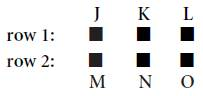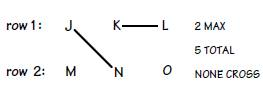# LRDI Practice Set

J, K, L, M, N, and O are square ski chalets of the same size, which are positioned in two straight rows as shown below:J is directly opposite M; K is directly opposite N; and L is directly opposite O. After a snowstorm, residents shovel a single continuous path that connects all of the chalets and meets the following conditions:

1. The path is composed of five straight segments, each of which directly connects exactly two of the chalets.
2. Each chalet is directly connected by a segment of the path to another chalet.
3. No chalet is directly connected by segments of the path to more than two other chalets.
4. No segment of the path crosses any other segment.
5. One segment of the path directly connects chalets J and N, and another segment directly connects chalets K and L.
1. Which one of the following statements could be true?
1. One segment of the path directly connects chalets M and K.
2. One segment of the path directly connects chalets M and L.
3. One segment of the path directly connects chalets M and O.
4. One segment of the path directly connects chalets J and K and another segment directly connects chalets K and M.
5. One segment of the path directly connects chalets O and L and another segment directly connects chalets O and N.
1. If one segment of the path directly connects chalets K and N, then the two chalets in which one of the following pairs must be directly connected to each other by a segment?
1. J and K
2. K and O
3. L and O
4. M and N
5. N and O
1. If a segment of the path directly connects chalets J and K, then the two chalets in which one of the following pairs must be directly connected to each other by a segment?
1. J and M
2. K and N
3. K and O
4. L and O
5. N and O
1. If one segment of the path directly connects chalets K and O, then which one of the following statements could be true?
1. Chalet J is directly connected to chalet M.
2. Chalet K is directly connected to chalet N.
3. Chalet L is directly connected to chalet O.
4. Chalet L is directly connected to exactly two chalets.
5. Chalet O is directly connected to exactly one chalet.
1. Which one of the following statements, if true, guarantees that one segment of the path directly connects chalets M and N?
1. One segment of the path directly connects chalets K and J.
2. One segment of the path directly connects chalets N and O.
3. One segment of the path directly connects chalet K and a chalet in row 2.
4. One segment of the path directly connects chalet L and a chalet in row 2.
5. One segment of the path directly connects chalet O and a chalet in row 1.
1. Which one of the following chalets cannot be directly connected by segments of the path to exactly two other chalets?
1. K
2. L
3. M
4. N
5. O
1. If no segment of the path directly connects any chalet in row 1 with the chalet in row 2 that is directly opposite it, then each of the following statements must be true EXCEPT:
1. A segment of the path directly connects chalets M and N.
2. A segment of the path directly connects chalets N and O.
3. Chalet L is directly connected to exactly one other chalet.
4. Chalet N is directly connected to exactly two other chalets.
5. Chalet O is directly connected to exactly two other chalets.

The Key Issue is:

1) What chalets can, must, or cannot be directly connected to what other chalets to form a single continuous path?

The Initial Setup:

You’re given a wonderful sketch in the opening paragraph, so by all means, use it. As you work with the rules, build them right into the given sketch. Here’s a reproduction of the diagram that was provided:The Rules:

There’s one very important rule stated in the opening paragraph, and you could have easily missed it. It’s stated that “a single continuous path . . . connects all of the chalets.” This means that one single line must connect all the chalets with no backtracks (that’s one of the implications of the word “continuous.”) So a single path will wind around touching all the chalets—very important.

1) The path is composed of exactly 5 segments, each of which runs between exactly two chalets.

2) This is a basic loophole closer; no chalet is left out of the path.

3) Each chalet is connected to at most two other chalets. This rule hints at the importance of the numbers aspect in the set. When any chalet is connected to two others, then it’s maxed out. Write “2 MAX” by the provided sketch to serve as a visual reminder.

4) During the course of winding around to each chalet, the path cannot ever cross itself. Write “none cross.”

5) These rules are very concrete, so build them right into the given sketch. Draw a line between J and N, and draw another one between K and L. Key Deductions: There is something that you should notice just from reviewing the master sketch. Because of Rules 4 and 5, the only chalets that can be connected with M are J and N (and whichever one is connected to M will be maxed out thanks to Rule 3).

The Final Visualization: Here’s the master sketch:The Questions:

1. (E)

Not much to do with a “could be true” question with no new information except check the choices. Way down at (E) we see O connected to L and O connected to N. All we have to do is connect M and J to get an acceptable single continuous path.

(A) through (D) all try to connect M with a chalet other than J or N, not possible.

2. (C)

Since N and K are connected, both are maxed out. M must always be connected to either J or N, so here M must be connected to J and J is maxed out. The only  chalet that is left to be connected to O is L. You have a single continuous path and everything is set, so it’s a pretty simple task to scan the choices looking for the pair that must be connected. It’s L and O, (C). (A), (B), (D), and (E) are all impossible.

3. (D)

J and K are now maxed out. By now you should be used to looking for a chalet to hook up with M, and here it’s N. That’s two chalets connected to N, so N is maxed out. Just like in Q. 19, L must be connected to O, choice (D). (A), (B), (C), and (E) are all impossible.

4. (A)

This stem (K to O) maxes out K. As usual, J or N will connect with M. K is maxed and L is off limits to J, M, and N thanks to Rule 4, so O must be the one that connects the two groups. You don’t know whether O is connected to J or N, so on to the choices. As long as N is the one connected to O, J could be connected to M and (A) could be true.

(B) No, K is maxed out and can’t be connected to N.

(C) This would max out O without connecting all of the chalets; a no-no.

(D) The only way for L to be connected to two chalets is if the second one is O (L’s path can’t cross the K-O path) which would max out O and not connect all  the chalets.

(E) No, O is the chalet that has to connect in some way to the J, M, N group, so O must be connected to two chalets (K and either J or N), not one.

5. (A)

We’ve seen this basic concept before. M can only be connected to either J or N, so in order to force M to connect with N, all we need to do is max out J. This is accomplished by connecting J with either chalet K or chalet O; the testmakers chose the former as choice (A). (B) through (E) all leave open the possibility that M is connected to J, not K.

6. (C)

This should have been obvious because of all the previous work with M. The thinking goes like this: M can only be connected to J or N. If M is connected to  both of them, J and N are both maxed out, leaving no way to connect all the chalets with a single continuous path. M can be connected to only one chalet (J OR N), not two, which is choice (C). You could have checked each choice, but that would have taken much longer. If you did have to try out the choices, you could have sped things up by using your previous work, like so:

(A) In Q. 4, we saw K connected to two chalets, so axe (A).

(B) In Q. 2 (and 3), we saw L connected to two chalets; eliminate (B).

(D) In Q. 2, N was connected to two chalets.

(E) In Q. 4, we saw that O had to be connected to exactly two chalets.

7. (B)

Here we’re told that no direct opposites are connected, which means no J to M, no K to N, and no L to O. Where to now? We’re used to connecting M to either J or N, so start there. Since M and J can’t be connected, it’s M and N (N is now maxed out). L and O are not compatible according to this stem, so O can be connected to only J or K. Actually in this case, O must be connected to J and K. Otherwise, there would be no way to get our single continuous path. Everything is set. Only (B) contradicts this arrangement. (A), (C), (D), and (E) all follow the new sketch perfectly

150 CAT LRDI sets all with Video Explanations FREE Trial